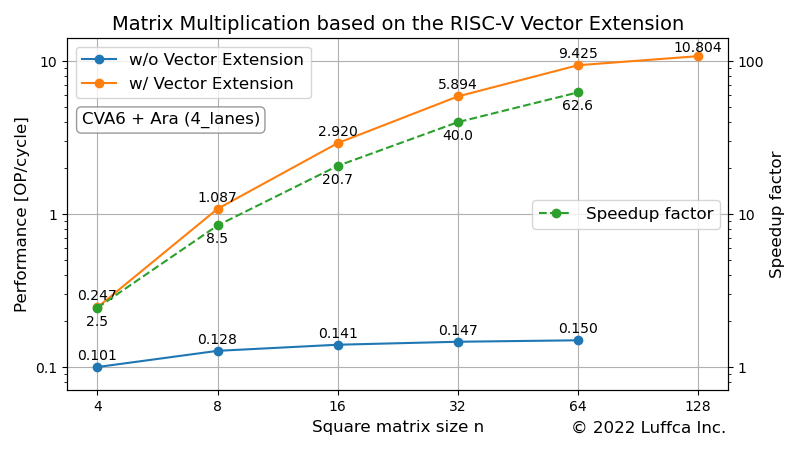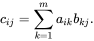# Matrix Multiplication based on the RISC-V Vector ExtensionWe have created a matrix multiplication kernel based on the RISC-V Vector Extension (RVV) and evaluated its performance using an RTL simulator.

## Matrix Multiplication

The matrix multiplication kernel computes an n × p matrix C, which is the product of an n × m matrix A and an m × p matrix B (C=AB). Let c_{ij} be the element of i-th row and j-th column of matrix C, and c_{ij} is expressed by the following formula:The code before vectorization looks like this:

```// C = AB with A = [n x m], B = [m x p], C = [n x p]
void matmul_int8(int32_t* c, const int8_t* a, const int8_t* b,
const unsigned long int n, const unsigned long int m,
const unsigned long int p) {
for (int i = 0; i < n; ++i) {
for (int j = 0; j < p; ++j) {
int32_t sum = 0;
for (int k = 0; k < m; ++k) {
sum += a[i * m + k] * b[k * p + j];
}
c[i * p + j] = sum;
}
}
}
```

Considering application to machine learning, the elements of matrix A and B are `int8_t`, and the elements of matrix C are `int32_t`.

In previous article, we tested LLVM/Clang auto-vectorization, but this time we created a matrix multiplication kernel by hand tuning.

## Ara

Ara is an implementation of the RISC-V Vector Extension developed by the Parallel Ultra Low Power (PULP) project. Ara’s repository has the following description:

Ara is a vector unit working as a coprocessor for the CVA6 core. It supports the RISC-V Vector Extension, version 1.0.

Ara ensures scalability by implementing a number of 64-bit vector unit called lane. The config directory contains `[2|4|8|16]_lanes.mk` for the Ara system configuration. Each lane contains an integer Arithmetic Logic Unit (ALU), an integer multiplier (MUL), and a Floating Point Unit (FPU) that can perform 64-bit wide integer and double precision floating point operations.

Note that the CVA6 in the quote is a 64-bit RISC-V core previously called PULP Ariane.

## Matrix Multiplication on Ara RTL Simulator

Ara can create an RTL simulator for Ara system using Verilator. This time, we used the default configuration `4_lanes.mk`.

Below is the console output when running our matrix multiplication kernel.

```\$ cd \$ARA/hardware
\$ app=imatmul_int8 make simv

...

=============
=  IMATMUL  =
=============

...

------------------------------------------------------------
Calculating a (32 x 32) x (32 x 32) matrix multiplication...
------------------------------------------------------------

Initializing matrices...
Calculating imatmul...
The execution took 11119 cycles.
The performance is 5.894055 OP/cycle.
Verifying result...
Passed.

------------------------------------------------------------
Calculating a (64 x 64) x (64 x 64) matrix multiplication...
------------------------------------------------------------

Initializing matrices...
Calculating imatmul...
The execution took 55627 cycles.
The performance is 9.425063 OP/cycle.
Verifying result...
Passed.
```

Compared to the number of cycles in CVA6, we achieved a 40x speedup for 32 x 32 matrix multiplication and a 62x speedup for 64 x 64 matrix multiplication.

## Summary

We have created a matrix multiplication kernel based on the RISC-V Vector Extension and evaluated its performance using Ara’s RTL simulator.

Previous article

Next article